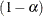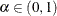# The SEVERITY Procedure

### Example 23.8 Predicting Mean and Value-at-Risk by Using Scoring Functions

If you work in the risk management department of an insurance company or a bank, then one of your primary applications of severity loss distribution models is to predict the value-at-risk (VaR), such that the probability of experiencing a loss value greater than the VaR is very low. The probability level at which VaR is measured is prescribed by industry regulations such as Basel III and Solvency II. The VaR level is usually specified in terms of, whereis the probability that a loss value exceeds the VaR. Typical VaR levels are 0.95, 0.975, and 0.995.

In addition to predicting VaR, which is regarded as an estimate of the worst-case loss, businesses are often interested in predicting the average loss by estimating either the mean or median of the distribution.

The estimation of the mean and VaR combined with the scale regression model is very potent tool for analyzing worst-case and average losses for various scenarios. For example, if the regressors that are used in a scale regression model represent some key macroeconomic and operational indicators, which are widely referred to as key risk indicators (KRIs), then you can analyze the VaR and mean loss estimates over various values for the KRIs to get a more comprehensive picture of the risk profile of your organization across various market and internal conditions.

This example illustrates the use of scoring functions to simplify the process of predicting the mean and VaR of scale regression models.

First, the following PROC FCMP steps define the functions to compute the mean for each of the 10 predefined distributions that are available in the Sashelp.Svrtdist library:

/*--------- Define distribution functions that compute the mean ----------*/
proc fcmp library=sashelp.svrtdist outlib=work.means.scalemod;
function BURR_MEAN(x, Theta, Alpha, Gamma);
if not(Alpha * Gamma > 1) then
return (.); /* first moment does not exist */
return (Theta*gamma(1 + 1/Gamma)*gamma(Alpha - 1/Gamma)/gamma(Alpha));
endsub;
function EXP_MEAN(x, Theta);
return (Theta);
endsub;
function GAMMA_MEAN(x, Theta, Alpha);
return (Theta*Alpha);
endsub;
function GPD_MEAN(x, Theta, Xi);
if not(Xi < 1) then
return (.); /* first moment does not exist */
return (Theta/(1 - Xi));
endsub;
function IGAUSS_MEAN(x, Theta, Alpha);
return (Theta);
endsub;
function LOGN_MEAN(x, Mu, Sigma);
return (exp(Mu + Sigma*Sigma/2.0));
endsub;

function PARETO_MEAN(x, Theta, Alpha);
if not(Alpha > 1) then
return (.); /* first moment does not exist */
return (Theta/(Alpha - 1));
endsub;
function STWEEDIE_MEAN(x, Theta, Lambda, P);
return (Theta* Lambda * (2 - P) / (P - 1));
endsub;
function TWEEDIE_MEAN(x, P, Mu, Phi);
return (Mu);
endsub;
function WEIBULL_MEAN(x, Theta, Tau);
return (Theta*gamma(1 + 1/Tau));
endsub;
quit;


The following statements include the Work.Means library in the CMPLIB= system option and submit a PROC SEVERITY step to estimate the scale regression models for various distributions by using a lognormal sample in the Work.Test_sev8 data set:

/*----- Fit all distributions and generate scoring functions ------*/
options cmplib=work.means;
proc severity data=test_sev8 outest=est print=all plots=none;
loss y;
scalemodel x1-x5;
dist _predefined_ stweedie;
outscorelib outlib=scorefuncs commonpackage;
run;


The SAS statements that simulate the sample in the Work.Test_sev8 data set are available in the PROC SEVERITY sample program sevex08.sas. The OUTLIB= option in the OUTSCORELIB statement requests that the scoring functions be written to the Work.Scorefuncs library, and the COMMONPACKAGE option in the OUTSCORELIB statement requests that all the functions be written to the same package. Upon completion, PROC SEVERITY sets the CMPLIB system option to the following value:

   (work.means sashelp.svrtdist work.scorefuncs)


The "All Fit Statistics" table in Output 23.8.1 shows that the lognormal distribution’s scale model is the best and the inverse Gaussian’s scale model is a close second according to the likelihood-based statistics.

Output 23.8.1: Comparison of Fitted Scale Models for Mean and VaR Illustration

The SEVERITY Procedure

All Fit Statistics
Distribution -2 Log
Likelihood
AIC AICC BIC KS AD CvM
stweedie 460.65755   476.65755   476.95083   510.37441   10.44548   64571   37.07705
Burr 451.42238   467.42238   467.71565   501.13924   10.32782   42254   37.19808
Exp 1515   1527   1527   1552   8.85827   29917   23.98267
Gamma 448.28222   462.28222   462.50986   491.78448   10.42272   63712   37.19450
Igauss 444.44512   458.44512   458.67276   487.94738   10.33028   83195   37.30880
Logn 444.43670 * 458.43670 * 458.66434 * 487.93895 * 10.37035   68631   37.18553
Pareto 1515   1529   1529   1559   8.85775 * 29916 * 23.98149 *
Gpd 1515   1529   1529   1559   8.85827   29917   23.98267
Weibull 527.28676   541.28676   541.51440   570.78902   10.48084   72814   36.36039

You can examine the scoring functions that are written to the Work.Scorefuncs library by using the FCMP Function Editor that is available in the Display Manager session of Base SAS when you select SolutionsAnalysis from the main menu. For example, PROC SEVERITY automatically generates and submits the following PROC FCMP statements to define the scoring functions 'SEV_MEAN_LOGN' and 'SEV_QUANTILE_IGAUSS':

proc fcmp library=(work.means sashelp.svrtdist) outlib=work.scorefuncs.sevfit;
function SEV_MEAN_LOGN(y, x{*});
_logscale_=0;
_logscale_ = _logscale_ + ( 7.64722278930350E-01 * x{1});
_logscale_ = _logscale_ + ( 2.99209540369860E+00 * x{2});
_logscale_ = _logscale_ + (-1.00788916253430E+00 * x{3});
_logscale_ = _logscale_ + ( 2.58883602184890E-01 * x{4});
_logscale_ = _logscale_ + ( 5.00927479793970E+00 * x{5});
_logscale_ = _logscale_ + ( 9.95078833050690E-01);
return (LOGN_MEAN(y, _logscale_,  2.31592981635590E-01));
endsub;

function SEV_QUANTILE_IGAUSS(y, x{*});
_logscale_=0;
_logscale_ = _logscale_ + ( 7.64581738373520E-01 * x{1});
_logscale_ = _logscale_ + ( 2.99159055015310E+00 * x{2});
_logscale_ = _logscale_ + (-1.00793496641510E+00 * x{3});
_logscale_ = _logscale_ + ( 2.58870460543840E-01 * x{4});
_logscale_ = _logscale_ + ( 5.00996884646730E+00 * x{5});
_scale_ =  2.77854870591020E+00 * exp(_logscale_);
return (IGAUSS_QUANTILE(y, _scale_,  1.81511227238720E+01));
endsub;
quit;


An important point to note is that the dist_MEAN distribution functions are not available in the original definition of any of the distributions in the Sashelp.Svrtdist library. PROC SEVERITY detects the availability of those functions in the Work.Means library that is included in the value of the CMPLIB= system option just before submitting the PROC SEVERITY step. Each dist_MEAN distribution function has a signature that matches the signature of a distribution function of the respective distribution, so PROC SEVERITY creates the corresponding scoring functions. Specifying the COMMONPACKAGE option in the OUTSCORELIB statement causes the name of the scoring function to take the form SEV_MEAN_dist. You can define any distribution function that has the desired signature to compute an estimate of your choice, include its library in the CMPLIB= system option, and then specify the OUTSCORELIB statement to generate the corresponding scoring functions.

To illustrate the use of scoring functions, let Work.Reginput contain the scoring data, where the values of regressors in each observation define one scenario. Scoring functions make it very easy to compute the mean and VaR of each distribution’s scale model for each of the scenarios, as the following steps illustrate for the lognormal and inverse Gaussian distributions:

/*--- Set VaR level ---*/
%let varLevel=0.975;

/*--- Compute scores (mean and var) for the       ---
--- scoring data by using the scoring functions ---*/
data scores;
array x{*} x1-x5;
set reginput;

igauss_mean = sev_mean_igauss(., x);
igauss_var  = sev_quantile_igauss(&varLevel, x);
logn_mean   = sev_mean_logn(., x);
logn_var    = sev_quantile_logn(&varLevel, x);
run;


The preceding steps use a VaR level of 97.5%.

The following DATA step accomplishes the same task by reading the parameter estimates that were written to the Work.Est data set by the previous PROC SEVERITY step:

/*--- Compute scores (mean and var) for the       ---
--- scoring data by using the OUTEST= data set  ---*/
data scoresWithOutest(keep=x1-x5 igauss_mean igauss_var logn_mean logn_var);
array _x_{*} x1-x5;
array _xparmIgauss_{5} _temporary_;
array _xparmLogn_{5} _temporary_;
retain _Theta0_ Alpha0;
retain _Mu0_ Sigma0;
*--- read parameter estimates for igauss and logn models ---*;
if (_n_ = 1) then do;
set est(where=(upcase(_MODEL_)='IGAUSS' and _TYPE_='EST'));
_Theta0_ = Theta; Alpha0 = Alpha;
do _i_=1 to dim(_x_);
if (_x_(_i_) = .R) then _xparmIgauss_(_i_) = 0;
else _xparmIgauss_(_i_) = _x_(_i_);
end;
set est(where=(upcase(_MODEL_)='LOGN' and _TYPE_='EST'));
_Mu0_ = Mu; Sigma0 = Sigma;
do _i_=1 to dim(_x_);
if (_x_(_i_) = .R) then _xparmLogn_(_i_) = 0;
else _xparmLogn_(_i_) = _x_(_i_);
end;
end;
set reginput;

*---  predict mean and VaR for inverse Gaussian  ---*;
* first compute X'*beta for inverse Gaussian *;
_xbeta_ = 0.0;
do _i_ = 1 to dim(_x_);
_xbeta_ = _xbeta_ + _xparmIgauss_(_i_) * _x_(_i_);
end;
* now compute scale for inverse Gaussian *;
_SCALE_ = _Theta0_ * exp(_xbeta_);
igauss_mean = igauss_mean(., _SCALE_, Alpha0);
igauss_var = igauss_quantile(&varLevel, _SCALE_, Alpha0);
*---  predict mean and VaR for lognormal         ---*;
* first compute X'*beta for lognormal*;
_xbeta_ = 0.0;
do _i_ = 1 to dim(_x_);
_xbeta_ = _xbeta_ + _xparmLogn_(_i_) * _x_(_i_);
end;
* now compute Mu=log(scale) for lognormal *;
_MU_ = _Mu0_ + _xbeta_;
logn_mean = logn_mean(., _MU_, Sigma0);
logn_var = logn_quantile(&varLevel, _MU_, Sigma0);
run;


The "Values Comparison Summary" table in Output 23.8.2 shows that the difference between the estimates that are produced by both methods is within the acceptable machine precision. However, the comparison of the DATA step complexity of each method clearly shows that the method that uses the scoring functions is much easier because it saves a lot of programming effort. Further, new distribution functions, such as the dist_MEAN functions that are illustrated here, are automatically discovered and converted to scoring functions by PROC SEVERITY. That enables you to focus your efforts on writing the distribution function that computes your desired score, which needs to be done only once. Then, you can create and use the corresponding scoring functions multiple times with much less effort.

Output 23.8.2: Comparison of Mean and VaR Estimates of Two Scoring Methods

  Observation Summary Observation Base Compare First Obs 1 1 Last Obs 10 10 Number of Observations in Common: 10. Total Number of Observations Read from WORK.SCORESWITHOUTEST: 10. Total Number of Observations Read from WORK.SCORES: 10. Number of Observations with Some Compared Variables Unequal: 0. Number of Observations with All Compared Variables Equal: 10. Values Comparison Summary Number of Variables Compared with All Observations Equal: 9. Number of Variables Compared with Some Observations Unequal: 0. Total Number of Values which Compare Unequal: 0. Total Number of Values not EXACTLY Equal: 40. Maximum Difference Criterion Value: 2.0963E-13.